T-LAB PLUS 2020 - ON-LINE HELP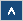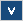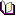T-LABIntroduction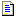What T-LAB does and what it enables us to doRequirements and PerformancesCorpus PreparationCorpus PreparationStructural CriteriaFormal CriteriaFileImport a single file...Prepare a Corpus (Corpus Builder)Open an existing projectSettingsAutomatic and Customized SettingsDictionary BuildingCo-occurrence AnalysisWord AssociationsCo-Word Analysis and Concept MappingComparison between Word pairsSequence and Network AnalysisConcordancesThematic AnalysisThematic Analysis of Elementary ContextsModeling of Emerging ThemesThematic Document ClassificationDictionary-Based ClassificationTexts and Discourses as Dynamic SystemsComparative AnalysisSpecificity AnalysisCorrespondence AnalysisMultiple Correspondence AnalysisCluster AnalysisSingular Value DecompositionLexical ToolsText Screening / DisambiguationsCorpus VocabularyStop-Word ListMulti-Word ListWord SegmentationOther ToolsVariable ManagerAdvanced Corpus SearchKey Contexts of Thematic WordsContingency TablesEditorImport-Export Identifiers listGlossaryAnalysis UnitAssociation IndexesChi-SquareCluster AnalysisCodingContext UnitCorpus and SubsetsCorrespondence AnalysisData TableDisambiguationDictionaryElementary ContextFrequency ThresholdGraph MakerHomographIDnumberIsotopyKey-Word (Key-Term)LemmatizationLexical UnitLexie and LexicalizationMarkov ChainMDSMultiwordsN-gramsNaïve BayesNormalizationOccurrences and Co-occurrencesPoles of FactorsPrimary DocumentProfileSpecificityStop Word ListTest ValueThematic NucleusTF-IDFVariables and CategoriesWords and LemmasBibliography
www.tlab.it

Chi-Square

Chi-square is a statistical test to check if the frequency values obtained by a survey and recorded in some cross-table, are significantly different from the theoretical ones (the "expected" values).

Generally, T-LAB applies this test to (2 x 2) tables and the threshold value is 3.84 (df = 1; p. 0.05) or 6.64 (df = 1; p. 0.01).

For example, in order to verify the significance of a word ("x") occurrences within a context unit ("A") the test is applied to a table as follows: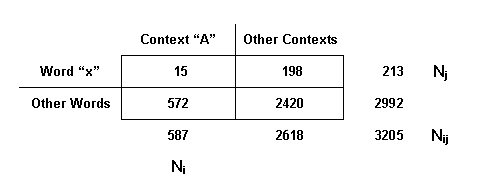The chi-square formula, in its simplified version, is the following: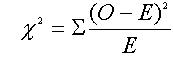where "O" and "E" stand respectively for the observed frequencies and the expected ones.

For each cell, the expected (E) occurrences are calculated as follows: (Ni x Nj)/Nij.

Following the above example the CHI value is equal to 19.38.

Since it is greater than the critical value, the null hypothesis (absence of meaningful difference) can be rejected.